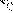# MINLPLib

### A Library of Mixed-Integer and Continuous Nonlinear Programming Instances

#### Removed Instance bearing

 Formatsⓘ ams gms mod nl osil py Primal Bounds (infeas ≤ 1e-08)ⓘ 1.95173322 p1 ( gdx sol ) (infeas: 3e-14)1.95168396 p2 ( gdx sol ) (infeas: 7e-10) Other points (infeas > 1e-08)ⓘ 1.93618060 p3 ( gdx sol ) (infeas: 5e-07)1.92826666 p4 ( gdx sol ) (infeas: 9e-07)1.91339147 p5 ( gdx sol ) (infeas: 9e-07) Dual Boundsⓘ 1.95173322 (ANTIGONE)1.90809929 (BARON)1.86055753 (COUENNE)1.95173322 (LINDO)1.94733263 (SCIP) Referencesⓘ Siddall, James N, Optimal Engineering Design: Principles and Applications, Marcel Dekker, New York, 1982.Deb, Kalyanmoy and Goyal, Mayank, Optimizing Engineering Designs Using a Combined Genetic Search. In Bäck, Thomas, Ed, Proceedings of the Seventh International Conference on Genetic Algorithms, 1997, 521-528.Coello Coello, Carlos A, Treating Constraints as Objectives for Single-Objective Evolutionary Optimization, Engineering Optimization, 32:3, 2000, 275-308. Sourceⓘ GAMS Model Library model bearing Applicationⓘ Hydrostatic Thrust Bearing Design Added to libraryⓘ 31 Jul 2001 Removed from libraryⓘ 16 Feb 2022 Removed becauseⓘ Difficult numerical behavior. Optimal value changes by > 2% when increasing feasibility tolerance. Problem typeⓘ NLP #Variablesⓘ 13 #Binary Variablesⓘ 0 #Integer Variablesⓘ 0 #Nonlinear Variablesⓘ 12 #Nonlinear Binary Variablesⓘ 0 #Nonlinear Integer Variablesⓘ 0 Objective Senseⓘ min Objective typeⓘ linear Objective curvatureⓘ linear #Nonzeros in Objectiveⓘ 2 #Nonlinear Nonzeros in Objectiveⓘ 0 #Constraintsⓘ 12 #Linear Constraintsⓘ 3 #Quadratic Constraintsⓘ 4 #Polynomial Constraintsⓘ 3 #Signomial Constraintsⓘ 0 #General Nonlinear Constraintsⓘ 2 Operands in Gen. Nonlin. Functionsⓘ log log10 vcpower Constraints curvatureⓘ indefinite #Nonzeros in Jacobianⓘ 38 #Nonlinear Nonzeros in Jacobianⓘ 28 #Nonzeros in (Upper-Left) Hessian of Lagrangianⓘ 32 #Nonzeros in Diagonal of Hessian of Lagrangianⓘ 6 #Blocks in Hessian of Lagrangianⓘ 2 Minimal blocksize in Hessian of Lagrangianⓘ 1 Maximal blocksize in Hessian of Lagrangianⓘ 11 Average blocksize in Hessian of Lagrangianⓘ 6.0 #Semicontinuitiesⓘ 0 #Nonlinear Semicontinuitiesⓘ 0 #SOS type 1ⓘ 0 #SOS type 2ⓘ 0 Minimal coefficientⓘ 6.0000e-06 Maximal coefficientⓘ 1.0965e+10 Infeasibility of initial pointⓘ 1.008e+05 Sparsity JacobianⓘSparsity Hessian of Lagrangianⓘ```\$offlisting
*
*  Equation counts
*      Total        E        G        L        N        X        C        B
*         13       10        1        2        0        0        0        0
*
*  Variable counts
*                   x        b        i      s1s      s2s       sc       si
*      Total     cont   binary  integer     sos1     sos2    scont     sint
*         14       14        0        0        0        0        0        0
*  FX      0
*
*  Nonzero counts
*      Total    const       NL      DLL
*         41       13       28        0
*
*  Solve m using NLP minimizing objvar;

Variables  x1,x2,x3,x4,objvar,x6,x7,x8,x9,x10,x11,x12,x13,x14;

Equations  e1,e2,e3,e4,e5,e6,e7,e8,e9,e10,e11,e12,e13;

e1..    10000*objvar - 10000*x7 - 10000*x8 =E= 0;

e2.. -1.42857142857143*x4*x6 + 10000*x8 =E= 0;

e3.. 10*x7*x9 - 0.00968946189201592*(x1**4 - x2**4)*x3 =E= 0;

e4.. 143.3076*x10*x4 - 10000*x7 =E= 0;

e5.. 3.1415927*(0.001*x9)**3*x6 - 6e-6*x3*x4*x13 =E= 0;

e6.. 101000*x12*x13 - 1.57079635*x6*x14 =E= 0;

e7.. log10(0.8 + 8.112*x3) - 10964781961.4318*x11**(-3.55) =E= 0;

e8..  - 0.5*x10 + x11 =E= 560;

e9..    x1 - x2 =G= 0;

e10.. 0.0307*sqr(x4) - 0.3864*sqr(0.0062831854*x1*x9)*x6 =L= 0;

e11..    101000*x12 - 15707.9635*x14 =L= 0;

e12.. -(log(x1) - log(x2)) + x13 =E= 0;

e13.. -(sqr(x1) - sqr(x2)) + x14 =E= 0;

* set non-default bounds
x1.lo = 1; x1.up = 16;
x2.lo = 1; x2.up = 16;
x3.lo = 1; x3.up = 16;
x4.lo = 1; x4.up = 16;
x6.lo = 1; x6.up = 1000;
x7.lo = 0.0001;
x8.lo = 0.0001;
x9.lo = 1;
x10.up = 50;
x11.lo = 100;
x12.lo = 1;
x13.lo = 0.0001;
x14.lo = 0.01;

* set non-default levels
x1.l = 6;
x2.l = 5;
x3.l = 6;
x4.l = 3;
x6.l = 1000;
x7.l = 1.6;
x8.l = 0.3;
x10.l = 50;
x11.l = 600;

Model m / all /;

m.limrow=0; m.limcol=0;
m.tolproj=0.0;

\$if NOT '%gams.u1%' == '' \$include '%gams.u1%'

\$if not set NLP \$set NLP NLP
Solve m using %NLP% minimizing objvar;

```

Last updated: 2023-06-01 Git hash: fdbb0df7